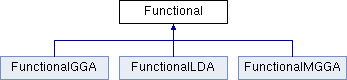JDFTx  1.7.0Functional Class Referenceabstract

Abstract base class for functionals. More...

#include <ExCorr_internal.h>

Inheritance diagram for Functional:## Public Member Functions

Functional (double scaleFac=1.0)

virtual bool needsSigma () const =0
return true if density gradients are used

virtual bool needsLap () const =0
return true if laplacian of density is used (MGGA)

virtual bool needsTau () const =0
return true if orbital kinetic energy density is used (MGGA)

virtual bool hasExchange () const =0
whether this functional includes exchange

virtual bool hasCorrelation () const =0
whether this functional includes correlation

virtual bool hasKinetic () const =0
whether this functional includes kinetic energy

virtual bool hasEnergy () const =0
whether total energy is meaningful for this functional

virtual void evaluate (int N, std::vector< const double * > n, std::vector< const double * > sigma, std::vector< const double * > lap, std::vector< const double * > tau, double *E, std::vector< double * > E_n, std::vector< double * > E_sigma, std::vector< double * > E_lap, std::vector< double * > E_tau) const =0

void evaluateSub (int iStart, int iStop, std::vector< const double * > n, std::vector< const double * > sigma, std::vector< const double * > lap, std::vector< const double * > tau, double *E, std::vector< double * > E_n, std::vector< double * > E_sigma, std::vector< double * > E_lap, std::vector< double * > E_tau) const
Call evaluate for a subset of data points:

## Protected Attributes

double scaleFac
scale factor (to support mixing for hybrid functionals)

## Detailed Description

Abstract base class for functionals.

## ◆ evaluate()

 virtual void Functional::evaluate ( int N, std::vector< const double * > n, std::vector< const double * > sigma, std::vector< const double * > lap, std::vector< const double * > tau, double * E, std::vector< double * > E_n, std::vector< double * > E_sigma, std::vector< double * > E_lap, std::vector< double * > E_tau ) const
pure virtual

Compute exchange-correlation energy densities and optionally gradients if E_n is non-null. Note that if E_n is non-null, then so must all components of E_n and E_sigma (if a GGA). All the energies and gradients must be scaled by scaleFac.

Parameters
 N number of points n (spin-)densities: 1 or 2 vectors for unpolarized / polarized sigma contracted (spin-)density gradient contractions: 1 or 3 vectors for unpolarized / polarized lap laplacian of (spin-)denisties tau kinetic energy density per psin channel (form orbitals) E accumulate energy density per volume E_n accumulate gradient w.r.t n's (if E_n is non-null) E_sigma accumulate gradient w.r.t sigma's (if E_n is non-null) E_lap accumulate gradient w.r.t lap's (if E_n is non-null) E_tau accumulate gradient w.r.t tau's (if E_n is non-null)

Implemented in FunctionalMGGA, FunctionalLDA, and FunctionalGGA.

The documentation for this class was generated from the following file: